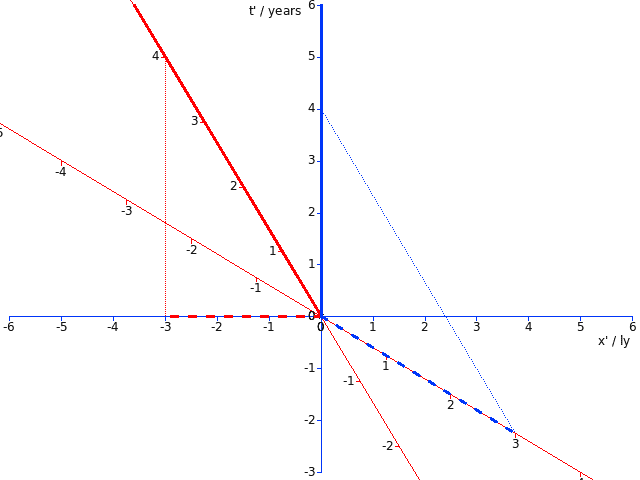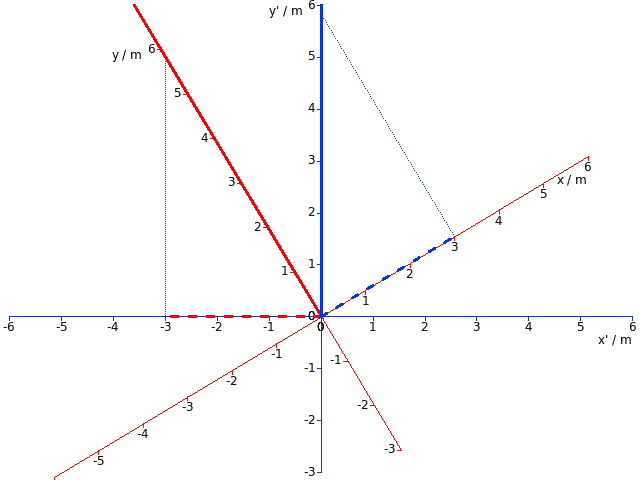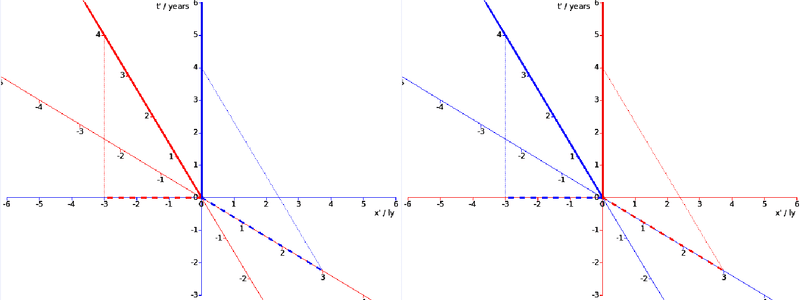# V=dx/dt (relative velocity between two inertial observers)

• B
• Kairos
Last edited:
••vanhees71, etotheipi and Ibix
If I am not wrong, this is precisely what the OP believes that you have been denying all through this thread!
No, I've given the general formula and always stressed in which special cases the naive relative velocity comes out and when the two relative velocities are opposite to each other, namely precisely when ##\vec{v}_1 \parallel \vec{v}_2## :-).

I thought I'd have a shot at some Minkowski diagrams, which may be helpful. I'm restricting motion to one dimension, but this is actually completely general for intersecting inertial worldlines, since in either one's rest frame we can simply define the x direction as "##\pm## the direction in which the other guy is moving". So these diagrams are all drawn in the hyperplane @etotheipi shaded in #30.

So we have two observers, red and blue. Here's a Minkowski diagram in red's frame - red's worldline is straight up the page and blue's is slanted because they're doing 0.6c.
View attachment 282015
Red can drop a perpendicular to the x-axis and draw blue's three-velocity, the heavy dotted blue line. This is what red calls "blue's velocity through space", so it must be a vector in the spatial direction. Blue's four-velocity is, of course, parallel to blue's worldline.

(Apologies that there's no arrowhead on the three-velocity - I didn't add the capability to draw arrowheads when I wrote my Minkowski diagram software.)

Now we can do the same thing in blue's frame:
View attachment 282016
Aside from the colours, this is an exact mirror image of the first diagram. This is the "reciprocity" between the red and blue's three-velocities that we've been talking about.

But the complexity comes in if we draw what red called "blue's velocity through space" on the diagram above:
View attachment 282017
I've included red's axes and the dropped perpendicular and three-velocity from the first diagram. And here we can see the problem with comparing three-velocities in different frames - three velocities in the red frame lie along the red spatial axis and three-velocities in the blue frame lie along the blue spatial axis. You can't compare them because they lie in different spatial planes. You can "promote" them to four-vectors (which I have kind of implicitly done by drawing them both on one diagram here), and then you can compare them, but they aren't parallel and boosting the blue dotted line to be parallel to the blue axis would not yield blue's three velocity in this frame (a problem @vanhees71 alluded to in #35).

So, the "reciprocity" of the three-velocities each observer assigns to the other in their respective rest frames just means that we can draw the first two Minkowski diagrams and they are mirror-symmetric. It follows from the symmetry of the situation - it must be true unless there's some difference between the red and blue observers, and there isn't. However, the three velocities don't lie in the same space and can't be meaningfully compared as vectors - you are, of course, free to compare their magnitudes.

Thank you for your diagrams. Your first two diagrams represent exactly what I was attempting to say in my post #8.. before the debate on the non reciprocity of relative velocity.

When you write "But the complexity comes in if we draw ..", do you draw an alternative representation of the same thing or of an another thing? supposedly a different configuration of inertial observers?

When you write "But the complexity comes in if we draw ..", do you draw an alternative representation of the same thing or of an another thing? supposedly a different configuration of inertial observers?
The third diagram is simply the second diagram with some extra lines copied and transformed from the first diagram. But it's clear from this that the three-velocities of each object in the rest frame of the other are not opposites, despite what the first two diagrams (or your intuition) might suggest.

The Euclidean diagrams are analogous - the x extent of one line and the x' extent of the other are reciprocal but not opposite.

Thank you, indeed it is difficult to fight against a strong intuition with mathematics. I'm not sure I grasp the meaning of opposite because 'reciprocal' means for me that the perception of the other is identical for each. One last attempt if you will: imagine a theoretical elastic thread (without restoring force) linking the two inertial objects. Do you agree with me that the rate of shortening or extension of this thread is necessarily reciprocal for both objects since it is unique? It seems to me that whatever the chosen reference frame, the relative velocity vector can be univocally defined as a function of this unique thread rate change.

It seems to me that whatever the chosen reference frame, the relative velocity vector can be univocally defined as a function of this unique thread rate change.
You can certainly define a three-velocity in each frame totally unambiguously, yes. The problem is that you have two reference frames with different definitions of space, so those three vectors don't lie in the same 3d subspace of 4d spacetime, so they cannot be compared (except comparing magnitudes, which are equal). You can see that in the third diagram, reproduced here:The red dotted line is "red's three-velocity according to blue" and it lies along the x' axis. The blue dotted line is "blue's three-velocity according to red" and it lies along the x axis. These axes are not parallel, so these vectors (treated as three vectors) do not exist in the same space, or ("promoted" to four vectors) are not parallel so aren't opposite.

The principle of relativity requires that if I measure your velocity relative to me to be ##v## then you must measure my velocity relative to you to be ##-v##. So yes, to answer the question in your post, we will agree that a thread connecting us would need to be payed out at the same rate. But since we don't agree what we mean by "space" that does not mean that we are measuring opposite velocities, oddly enough.

The Euclidean analogy is useful. I reproduced the analogous diagram below:Here the red dotted line is the blue frame's notion of the x'-component of a vector along the solid red line, and the blue dotted line is the red frame's notion of the x-component of a vector along the solid blue line. These components are the same length, but they aren't opposites because the directions they lie in are rotated with respect to one another.

Hope that makes sense.

•Kairos, vanhees71 and PeterDonis
Very clear, thank you. I wonder if the origin of the misunderstanding is that we didn't agree on a mathematical definition of reciprocity from the beginning. My view of reciprocity is illustrated below using one of your diagrams and simply swapping blue and red:The points of view of A and B are the same, not opposite but just the same. I believe that this definition of reciprocity in this context allows that, if A and B are both sources and observers, they see exactly the same Doppler effects.
I don't know if there is an official definition of reciprocity but from your considerations on opposite diagrams, I have the impression that you are rather talking about symmetry. By construction of the SR spacetime, everything depends on the point of view (except the spacetime intervals between events), so one cannot expect a single symmetric diagram for A and B if the viewpoint is asymmetric with respect to the system A,B. But let's introduce a third inertial observer standing forever in the middle of the elastic thread postulated above (#75). Seen from his reference frame, A and B would have symmetric spacetime trajectories on a single diagram.